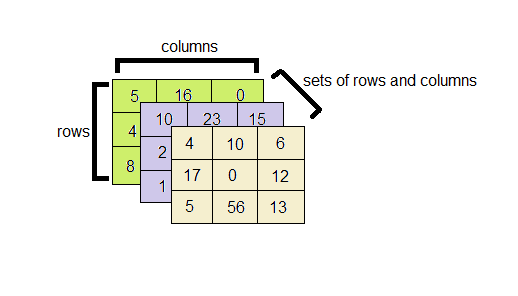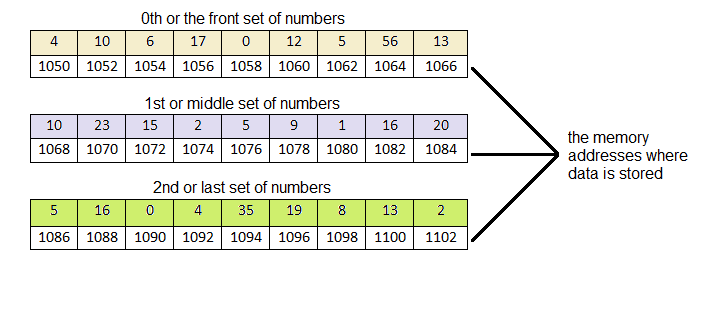# 3D Arrays in C language – How to declare, initialize and access elements

In our previous tutorials we have discussed that C programming allows multiple dimensions in arrays like 1D arrays2D arrays. Similarly, we can have three or more dimensions too. A 3D array is like a three dimensional figure ,eg: a cube or a cuboid.

A 3D array has rows and columns like a 2D array and another dimension which contains sets of these 2D rows and columns. The following figure illustrates the three dimensions of a 3D array:In this figure, we have an integer array. We have sets of numbers present in the rows and columns of this array. Like a two dimensional array, the representation of dimensions is done as 3x3x3. Where the numbers are – number of the row and column set * number of rows * number of column. We can have different values of all the dimensions like 2x3x2 or 4x3x3. To have a better understanding of how the data is stored in the memory for a 3D array, have a look at the explained figure of the array mentioned above:The above figure shows memory mapping of a 3D array. The consecutive memory addresses differ by 2 because the size of the int data type is 2 bytes. The above memory address has been taken randomly. In reality, the processor can store the array in other memory locations too. We also see that the numbers are stored in a linear fashion in the given array. In the upcoming sections, we shall learn more about the declaration, accessing and displaying the data in a 3D array.

### Declaration, Initialization and storing data

The declaration of a 3D array takes place in a very similar manner to that of any other array, like 1D or 2D array. A datatype is given to array to specify how the data in the array should be interpreted. Here is the syntax for declaration of an array:

int arr;

The int specifies that the data stored in the array will be of integer type.
arr is the variable name under which all the data is stored.
The first  refers to the sets of rows and columns of the array, the second  refers to the number of rows of the inner array and the third  refers to the number of columns of the inner array. This is also static memory allocation, that is, we are allocating the array a size equal to 3x3x3 , that is, the array can store 3x3x3 = 27 number of elements. The three [][][] brackets specifies the array is three dimensional.

Initialization of a 3D array needs to be done as follows:

```//the following syntax is used for proper readability
int arr={{       //set 1
{4,10,6},
{17,0,12},
{5,56,13}
},
{      //set 2
{10,23,15},
{2,5,9},
{1,16,20}
},
{      //set 3
{5,16,0},
{4,35,19},
{8,13,2}}};
```

Here we have initialized an integer array. Array of any type can be initialized using this format.

We already know that data can be stored in an array using for loops. Since we have a 3D array, we use 3 for loops for that purpose. Here is the syntax for storing data in a 3D array:

```for(i=0 ;i < 3 ;i++){
for(j=0 ;j < 3 ;j++){
for(k=0 ;k < 3 ;k++){
scanf("%d",&arr[i][j][k]);
}}}
```

Note that if we do not initialize the array or store data in it by user input, it will result in an output of garbage values.

### Accessing and Reading an array

We are already familiar with the concept of accessing the array using subscripts or index numbers in 2D arrays. Accessing 3D array is done in a very similar manner. The data present in row 2, column 1 and 2nd set is referred to as:

arr;

For the purpose of reading all the elements of the array, we use three nested for loops. This syntax is given below:

```for(i=0 ;i < 3 ;i++){ //the outer loop is for the set of rows and columns
for(j=0 ;j < 3 ;j++){ //this middle loop is for the rows
for(k=0 ;k < 3 ;k++){ //this inner loop is for the columns
printf("%d ",arr[i][j][k]);
}printf("\n");
}printf("\n");
}
```

### Program to initialize 3D array with User input, process and print it

Here is a simple program of 3D array which adds two arrays and stores the result in another array. One array has already been initialized and the other one will have data input by the user.

```
#include <stdio.h>
int main() {
// the first array has been initialized.
int a = {{// set 1
{4, 10, 6},
{17, 0, 12},
{5, 56, 13}},
{// set 2
{10, 23, 15},
{2, 5, 9},
{1, 16, 20}},
{// set 3
{5, 16, 0},
{4, 35, 19},
{8, 13, 2}}};
// second array shall have user input values and third array stores the sum of
// the other two
int b, c;
int i, j, k;

// input elements into the second array
printf("Enter the elements in the array:\n");

for (i = 0; i < 3; i++) {
for (j = 0; j < 3; j++) {
for (k = 0; k < 3; k++) {
scanf("%d", &b[i][j][k]);
c[i][j][k] = a[i][j][k] + b[i][j][k]; // summing up the two arrays
}
}
}

printf("The sum of the two arrays : \n");

for (i = 0; i < 3; i++) { // the outer loop is for the set of rows and columns
for (j = 0; j < 3; j++) {   // this middle loop is for the rows
for (k = 0; k < 3; k++) { // this inner loop is for the columns
printf("%d   ", c[i][j][k]);
}
printf("\n");
}
printf("\n");
}

return 0;
}

```
```Output:-
Enter the elements in the array:
6 7 34
4 9 6
0 2 3

18 9 7
13 4 0
1 23 65

8 6 3
9 3 5
3 15 32
The sum of the two arrays:
10  17  40
21  9  18
5  58  16

28  32  22
15  9  9
2  39  85

13  22  3
13  38  24
11  28  34
```

Learning never exhausts the mind.So, do come back for more. Hope this helps and you like the tutorial. Do ask for any queries in the comment box and provide your valuable feedback.

Share and subscribe.

Keep Coding!! Happy Coding!! 🙂

### Recommended -

Subscribe
Notify of